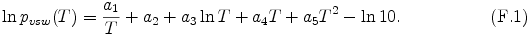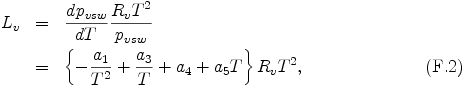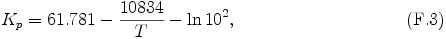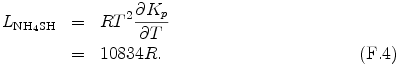## F. Calculation of Thermodynamical Properties

### Saturation vapor pressure and latent heat of H2O and NH3

#### Saturation vapor pressure

The expression of saturation vapor pressure pvsw is adopted from eq. (14) of Briggs and Sacket (1989) .The coefficients a1 - a5 in eq. (F.1) are given by Table F.1. Note that the term -ln10 is added from the expressoin of Briggs and Sacket (1989) , since SI units are used instead of cgs units.

#### Latent Heat

The latent heat per mole Lv is represented by using Clausius-Clapeyron equation and eq. (F.1) as follows.where Rv is the gas constant of each condensible species.

Table F.1: The coefficients in eqs. (F.1) and (F.2) (Briggs and Sacket, 1989 ).

Species a1 a a3 a4 a5
NH3(s) -4122 41.67871 -1.81630 0 0
H2O(s) -5631.1206 -8.363602 8.2312 -3.861449 × 10-2 2.77494 × 10-5

### Equilibrium constant and reaction heat of NH4SH

#### Equilibrium constant

The equilibrium constant Kp in the NH4SH production reaction, H2S(g) + NH3(g) ↔ NH4SH(s), is adopted from eq. (19) of Briggs and Sacket (1989) .The term -ln102 has been added since SI units are used instead of cgs units.

#### Reaction Heat

The reaction heat per unit mol LNH4SH is represented by using the van't Hoff equation and eq. (F.3) as follows.where R is universal gas constant.